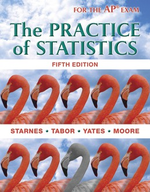×
Get Full Access to Statistics - Textbook Survival Guide
Get Full Access to Statistics - Textbook Survival Guide

×

Textbooks / Statistics / The Practice of Statistics 5

# The Practice of Statistics 5th Edition - Solutions by Chapter## Full solutions for The Practice of Statistics | 5th Edition

ISBN: 9781464108730The Practice of Statistics | 5th Edition - Solutions by Chapter

Solutions by Chapter
4 5 0 258 Reviews
##### ISBN: 9781464108730

The full step-by-step solution to problem in The Practice of Statistics were answered by , our top Statistics solution expert on 03/19/18, 03:52PM. This expansive textbook survival guide covers the following chapters: 44. Since problems from 44 chapters in The Practice of Statistics have been answered, more than 311696 students have viewed full step-by-step answer. This textbook survival guide was created for the textbook: The Practice of Statistics, edition: 5. The Practice of Statistics was written by and is associated to the ISBN: 9781464108730.

Key Statistics Terms and definitions covered in this textbook
• 2 k p - factorial experiment

A fractional factorial experiment with k factors tested in a 2 ? p fraction with all factors tested at only two levels (settings) each

• Analysis of variance (ANOVA)

A method of decomposing the total variability in a set of observations, as measured by the sum of the squares of these observations from their average, into component sums of squares that are associated with speciic deined sources of variation

• Bimodal distribution.

A distribution with two modes

• C chart

An attribute control chart that plots the total number of defects per unit in a subgroup. Similar to a defects-per-unit or U chart.

• Central limit theorem

The simplest form of the central limit theorem states that the sum of n independently distributed random variables will tend to be normally distributed as n becomes large. It is a necessary and suficient condition that none of the variances of the individual random variables are large in comparison to their sum. There are more general forms of the central theorem that allow ininite variances and correlated random variables, and there is a multivariate version of the theorem.

• Chi-square (or chi-squared) random variable

A continuous random variable that results from the sum of squares of independent standard normal random variables. It is a special case of a gamma random variable.

• Conditional mean

The mean of the conditional probability distribution of a random variable.

• Conidence level

Another term for the conidence coeficient.

• Continuous random variable.

A random variable with an interval (either inite or ininite) of real numbers for its range.

• Correlation coeficient

A dimensionless measure of the linear association between two variables, usually lying in the interval from ?1 to +1, with zero indicating the absence of correlation (but not necessarily the independence of the two variables).

• Correlation matrix

A square matrix that contains the correlations among a set of random variables, say, XX X 1 2 k , ,…, . The main diagonal elements of the matrix are unity and the off-diagonal elements rij are the correlations between Xi and Xj .

• Cumulative normal distribution function

The cumulative distribution of the standard normal distribution, often denoted as ?( ) x and tabulated in Appendix Table II.

• Curvilinear regression

An expression sometimes used for nonlinear regression models or polynomial regression models.

• Dispersion

The amount of variability exhibited by data

• Empirical model

A model to relate a response to one or more regressors or factors that is developed from data obtained from the system.

• Error mean square

The error sum of squares divided by its number of degrees of freedom.

• Expected value

The expected value of a random variable X is its long-term average or mean value. In the continuous case, the expected value of X is E X xf x dx ( ) = ?? ( ) ? ? where f ( ) x is the density function of the random variable X.

• Experiment

A series of tests in which changes are made to the system under study

• Factorial experiment

A type of experimental design in which every level of one factor is tested in combination with every level of another factor. In general, in a factorial experiment, all possible combinations of factor levels are tested.

• Gamma random variable

A random variable that generalizes an Erlang random variable to noninteger values of the parameter r